# Forecasting Markets using eXtreme Gradient Boosting (XGBoost)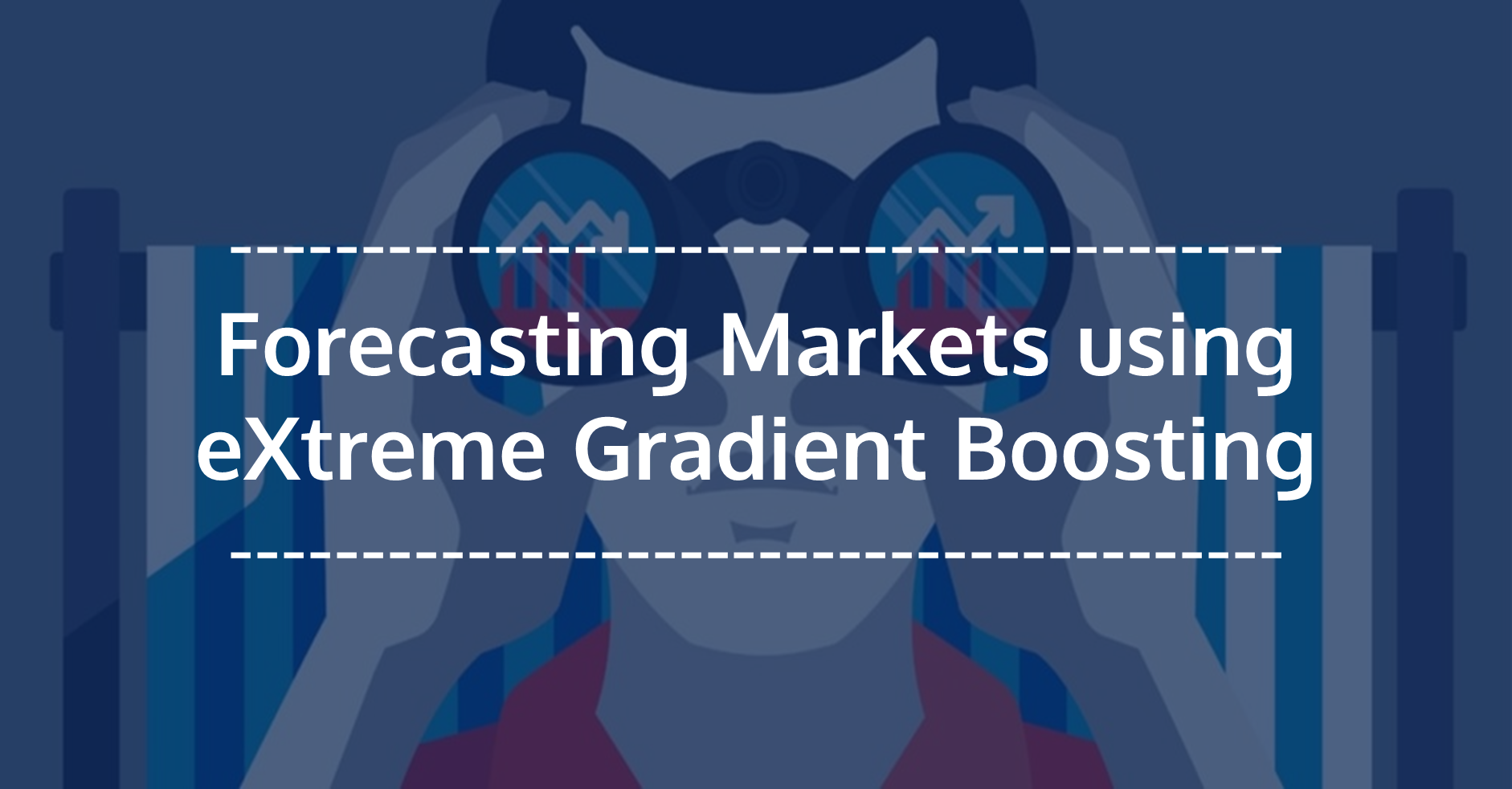In recent years, machine learning for trading has been generating a lot of curiosity for its profitable application to trading. Numerous machine learning models like Linear/Logistic regression, Support Vector Machines, Neural Networks, Tree-based models etc. are being tried and applied in an attempt to analyze and forecast the markets. Researchers have found that some models have more success rate compared to other machine learning models. eXtreme Gradient Boosting also called XGBoost is one such machine learning model that has attracted a lot of attention from the machine learning practitioners.

In this post, we will cover the basics of XGBoost, a winning model for many kaggle competitions. We then attempt to develop an XGBoost stock forecasting model using the “xgboost” package in R programming.

### Basics of XGBoost and related concepts

Developed by Tianqi Chen, the eXtreme Gradient Boosting (XGBoost) model is an implementation of the gradient boosting framework. Gradient Boosting algorithm is a machine learning technique used for building predictive tree-based models. (Machine Learning: An Introduction to Decision Trees).

Boosting is an ensemble technique in which new models are added to correct the errors made by existing models. Models are added sequentially until no further improvements can be made.

The ensemble technique uses the tree ensemble model which is a set of classification and regression trees (CART). The ensemble approach is used because a single CART, usually, does not have a strong predictive power. By using a set of CART (i.e. a tree ensemble model) a sum of the predictions of multiple trees is considered.

Gradient boosting is an approach where new models are created that predict the residuals or errors of prior models and then added together to make the final prediction.

The objective of the XGBoost model is given as:

Obj = L +

Where, L is the loss function which controls the predictive power, and Ω is regularization component which controls simplicity and overfitting

The loss function (L) which needs to be optimized can be Root Mean Squared Error for regression, Logloss for binary classification, or mlogloss for multi-class classification.

The regularization component (Ω) is dependent on the number of leaves and the prediction score assigned to the leaves in the tree ensemble model.

It is called gradient boosting because it uses a gradient descent algorithm to minimize the loss when adding new models. The Gradient boosting algorithm supports both regression and classification predictive modeling problems.### Sample XGBoost model:

We will use the “xgboost” R package to create a sample XGBoost model. You can refer to the documentation of the “xgboost” package here.

Install and load the xgboost library –

We install the xgboost library in R using the install.packages function. To load this package we use the library function. We also load other relevant packages required to run the code.

```install.packages("xgboost")# Load the relevant libraries
library(quantmod); library(TTR); library(xgboost);```

Create the input features and target variable – We take the 5-year OHLC and volume data of a stock and compute the technical indicators (input features) using this dataset. The indicators computed include Relative Strength Index (RSI), Average Directional Index (ADX), and Parabolic SAR (SAR). We create a lag in the computed indicators to avoid the look-ahead bias. This gives us our input features for building the XGBoost model in R. Since this is a sample model, we have included only a few indicators to build our set of input features.

```# Read the stock data
symbol = "ACC"
fileName = paste(getwd(),"/",symbol,".csv",sep="") ;
colnames(df) = c("Date","Time","Close","High", "Low", "Open","Volume")# Define the technical indicators to build the model
rsi = RSI(df\$Close, n=14, maType="WMA")
sar = SAR(df[,c("High","Low")], accel = c(0.02, 0.2))
trend = df\$Close - sar# create a lag in the technical indicators to avoid look-ahead bias

Our objective is to predict the direction of the daily stock price change (Up/Down) using these input features. This makes it a binary classification problem. We compute the daily price change and assigned a positive 1 if the daily price change is positive. If the price change is negative, we assign a zero value.

```# Create the target variable
price = df\$Close-df\$Open
class = ifelse(price > 0,1,0)```

Combine the input features into a matrix – The input features and the target variable created in the above step are combined to form a single matrix. We use the matrix structure in the XGBoost model since the xgboost libraryin R allows data in the matrix format.

```# Create a Matrix
model = na.omit(model)

Split the dataset into training data and test data – In the next step, we split the dataset into training and test data. Using this training and test dataset we create the respective input features set and the target variable.

```# Split data into train and test sets
train_size = 2/3
breakpoint = nrow(model) * train_size

training_data = model[1:breakpoint,]
test_data = model[(breakpoint+1):nrow(model),]# Split data training and test data into X and Y
X_train = training_data[,2:4] ; Y_train = training_data[,1]
class(X_train); class(Y_train)

X_test = test_data[,2:4] ; Y_test = test_data[,1]
class(X_test); class(Y_test)```

Train the XGBoost model on the training dataset –

We use the xgboost R function to train the model. The arguments of the xgboost R function are shown in the picture below.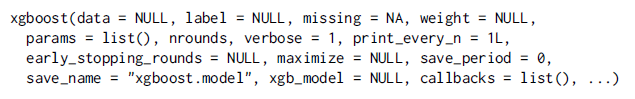The data argument in the xgboost R function is for the input features dataset. It accepts a matrix, dgCMatrix, or local data file. The nrounds argument refers to the max number of iterations (i.e. the number of trees added to the model). The obj argument refers to the customized objective function. It returns gradient and second order gradient with given prediction and dtrain.

```# Train the xgboost model using the "xgboost" function
dtrain = xgb.DMatrix(data = X_train, label = Y_train)
xgModel = xgboost(data = dtrain, nround = 5, objective = "binary:logistic")```

Output – The output is the classification error on the training data set.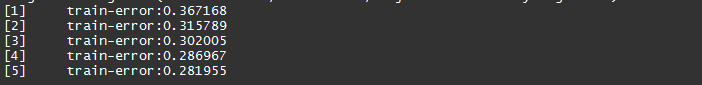Cross-validation

We can also use the cross-validation function of xgboost R i.e. xgb.cv. In this case, the original sample is randomly partitioned into nfold equal size subsamples. Of the nfold subsamples, a single subsample is retained as the validation data for testing the model, and the remaining (nfold - 1) subsamples are used as training data. The cross-validation process is then repeated nrounds times, with each of the nfold subsamples used exactly once as the validation data.

```# Using cross validation
dtrain = xgb.DMatrix(data = X_train, label = Y_train)
cv = xgb.cv(data = dtrain, nround = 10, nfold = 5, objective = "binary:logistic")```

Output - The xgb.cv returns a data.table object containing the cross validation results.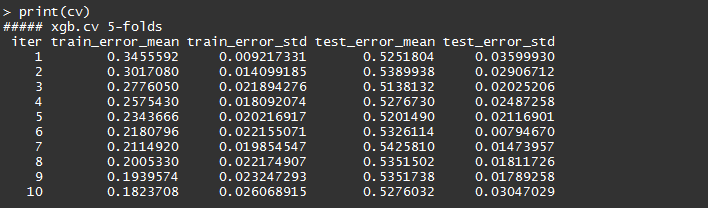Make predictions on the test data

To make predictions on the unseen data set (i.e. the test data), we apply the trained XGBoost model on it which gives a series of numbers.

```# Make the predictions on the test data
preds = predict(xgModel, X_test)# Determine the size of the prediction vector
print(length(preds))# Limit display of predictions to the first 6

Output –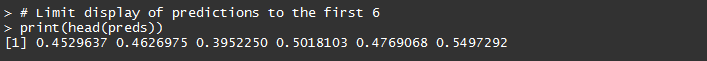These numbers do not look like binary classification {0, 1}. We have to, therefore, perform a simple transformation before we are able to use these results. In the example code shown below, we are comparing the predicted number to the threshold of 0.5. The threshold value can be changed depending upon the objective of the modeler, the metrics (e.g. F1 score, Precision, Recall) that the modeler wants to track and optimize.

```prediction = as.numeric(preds > 0.5)

Output -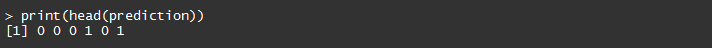Measuring model performance

Different evaluation metrics can be used to measure the model performance. In our example, we will compute a simple metric, the average error. It compares the predicted score with the threshold of 0.50.

For example: If the predicted score is less than 0.50, then the (preds > 0.5) expression gives a value of 0. If this value is not equal to the actual result from the test data set, then it is taken as a wrong result.

We compare all the preds with the respective data points in the Y_test and compute the average error. The code for measuring the performance is given below. Alternatively, we can use hit rate or create a confusion matrix to measure the model performance.

```# Measuring model performance
error_value = mean(as.numeric(preds > 0.5) != Y_test)
print(paste("test-error=", error_value))```

Output -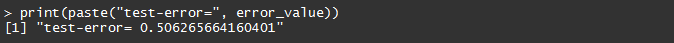Plot the feature importance set - We can find the top important features in the model by using the xgb.importance function.

```# View feature importance from the learnt model
importance_matrix = xgb.importance(model = xgModel)
print(importance_matrix)```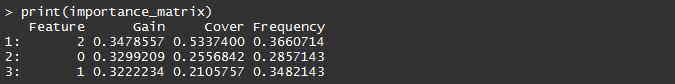Plot the XGBoost Trees

Finally, we can plot the XGBoost trees using the xgb.plot.tree function. To limit the plot to a specific number of trees, we can use the n_first_tree argument. If NULL, all trees of the model are plotted.

```# View the trees from a model
xgb.plot.tree(model = xgModel)# View only the first tree in the XGBoost model
xgb.plot.tree(model = xgModel, n_first_tree = 1)```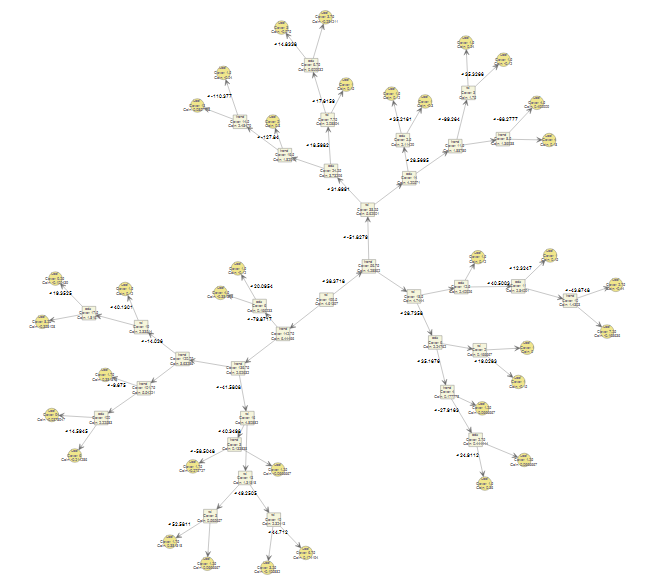### Conclusion

This post covered the popular XGBoost model along with a sample code in R programming to forecast the daily direction of the stock price change. Readers can catch some of our previous machine learning blogs (links given below). We will be covering more machine learning concepts and techniques in our coming posts.

### Next Step

Read our post on 'Forecasting Stock Returns Using ARIMA Model' that covers the popular ARIMA forecasting model to predict returns on a stock and demonstrate a step-by-step process of ARIMA modeling using R programming.

Disclaimer: All investments and trading in the stock market involve risk. Any decisions to place trades in the financial markets, including trading in stock or options or other financial instruments is a personal decision that should only be made after thorough research, including a personal risk and financial assessment and the engagement of professional assistance to the extent you believe necessary. The trading strategies or related information mentioned in this article is for informational purposes only.